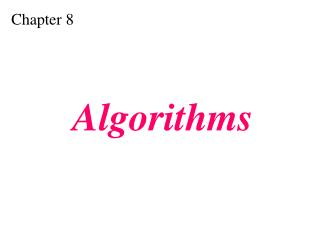DownloadDownload PresentationChapter 8

# Chapter 8

Télécharger la présentation## Chapter 8

- - - - - - - - - - - - - - - - - - - - - - - - - - - E N D - - - - - - - - - - - - - - - - - - - - - - - - - - -
##### Presentation Transcript

1. Chapter 8 Algorithms

2. OBJECTIVES Define and use the three constructs for developingalgorithms: sequence, decision, and repetition. Understand and use three tools to represent algorithms:flowchart, pseudocode, and structure chart. Understand the concept of modularity and subalgorithms. List and comprehend common algorithms. Understand the concept of an algorithm. After reading this chapter, the reader should be able to:

3. 8.1 CONCEPT

4. Figure 8-1 Informal definition of an algorithm used in a computer

5. Figure 8-2 Finding the largest integer among five integers

6. Figure 8-3 Defining actions in FindLargest algorithm

7. Figure 8-4 FindLargest refined

8. Figure 8-5 Generalization of FindLargest

9. 8.2 THREE CONSTRUCTS

10. Figure 8-6 Three constructs

11. 8.3 ALGORITHM REPRESENTATION

12. Figure 8-7 Flowcharts for three constructs

13. Figure 8-8 Pseudocode for three constructs

14. Example 1 Write an algorithm in pseudocode that finds the average of two numbers Solution See Algorithm 8.1 on the next slide.

15. Algorithm 8.1: Average of two • AverageOfTwo • Input:Two numbers • Add the two numbers • Divide the result by 2 • Return the result by step 2 • End

16. Example 2 Write an algorithm to change a numeric grade to a pass/no pass grade. Solution See Algorithm 8.2 on the next slide.

17. Algorithm 8.2: Pass/no pass Grade • Pass/NoPassGrade • Input:One number • if(the number is greater than or equal to 70)then • 1.1 Set the grade to “pass” • else • 1.2 Set the grade to “nopass” • End if • Return the grade • End

18. Example 3 Write an algorithm to change a numeric grade to a letter grade. Solution See Algorithm 8.3 on the next slide.

19. Algorithm 8.3: Letter grade • LetterGrade • Input:One number • 1. if(the number is between 90 and 100, inclusive)then • 1.1 Set the grade to “A” • End if • 2. if(the number is between 80 and 89, inclusive)then • 2.1 Set the grade to “B” • End if Continues on the next slide

20. Algorithm 8.3: Letter grade (continued) • 3. if(the number is between 70 and 79, inclusive)then • 3.1 Set the grade to “C” • End if • 4. if(the number is between 60 and 69, inclusive)then • 4.1 Set the grade to “D” • End if Continues on the next slide

21. Algorithm 8.3: Letter grade (continued) • 5. If(the number is less than 60)then • 5.1 Set the grade to “F” • End if • 6. Return the grade • End

22. Example 4 Write an algorithm to find the largest of a set of numbers. You do not know the number of numbers. Solution See Algorithm 8.4 on the next slide.

23. Algorithm 8.4: Find largest • FindLargest • Input:A list of positive integers • Set Largest to 0 • while (more integers) 2.1 if (the integer is greater than Largest) then 2.1.1 Set largest to the value of the integer End ifEnd while • Return Largest • End

24. Example 5 Write an algorithm to find the largest of 1000 numbers. Solution See Algorithm 8.5 on the next slide.

25. Algorithm 8.5: Find largest of 1000 numbers • FindLargest • Input:1000 positive integers • Set Largest to 0 • Set Counter to 0 • while (Counter less than 1000) 3.1 if (the integer is greater than Largest) then 3.1.1 Set Largest to the value of the integer End if 3.2 Increment CounterEnd while • Return Largest • End

26. 8.4 • MORE FORMA DEFINITION • Ordered set • Unambiguous steps • Effectiveness • Termination

27. 8.5 SUBALGORITHMS

28. Figure 8-9 Concept of a subalgorithm

29. Algorithm 8.6: Find largest • FindLargest • Input:A list of positive integers • Set Largest to 0 • while (more integers) 2.1 FindLargerEnd while • Return Largest • End

30. Subalgorithm: Find larger • FindLarger • Input:Largest and current integer • if (the integer is greater than Largest)then 1.1 Set Largest to the value of the integerEnd ifEnd

31. 8.6 BASIC ALGORITHMS

32. Figure 8-10 Summation

33. Figure 8-11 Product

34. Figure 8-12 Selection sort

35. Figure 8-13: part I Example of selection sort

36. Figure 8-13: part II Example of selection sort

37. Figure 8-14 Selection sort algorithm

38. Figure 8-15 Bubble sort

39. Figure 8-16: part I Example of bubble sort

40. Figure 8-16: part II Example of bubble sort

41. Figure 8-17 Insertion sort

42. Figure 8-18: part I Example of insertion sort

43. Figure 8-18: part II Example of insertion sort

44. Figure 8-19 Search concept

45. Figure 8-20: Part I Example of a sequential sort

46. Figure 8-20: Part II Example of a sequential sort

47. Example of a binary sort Figure 8-21

48. 8.1 RECURSION

49. Figure 8-22 Iterative definition of factorial

50. Figure 8-23 Recursive definition of factorial